*
1 point*
1 point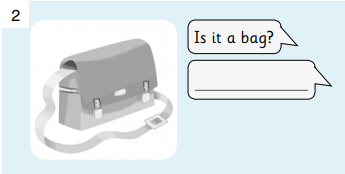*
1 point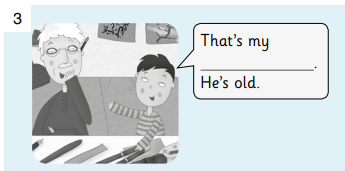*
1 point*
1 point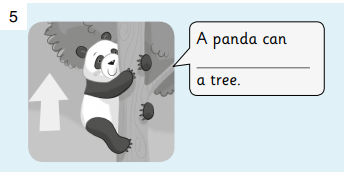*
1 point*
1 point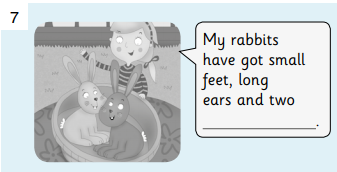*
1 point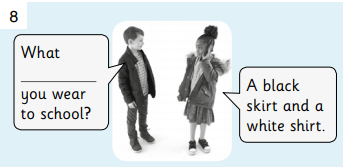*
1 point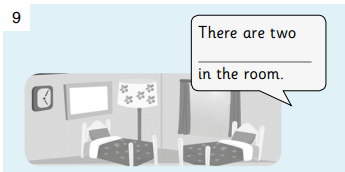*
1 point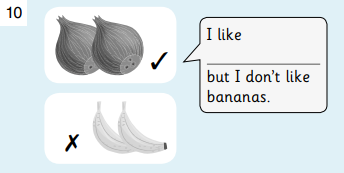*
1 point*
1 point*
1 point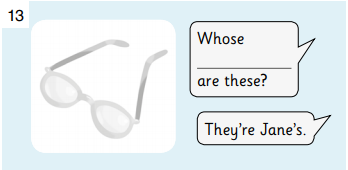*
1 point*
1 point*
1 point*
1 point*
1 point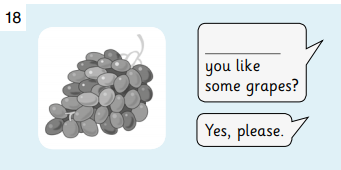*
1 point*
1 point*
1 point*
1 point*
1 point*
1 point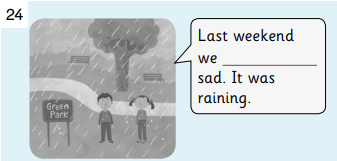*
1 point*
1 point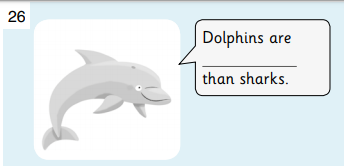*
1 point*
1 point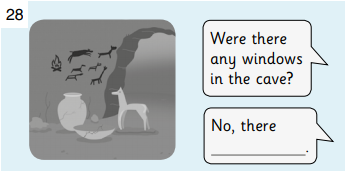*
1 point*
1 point*
1 point*
1 point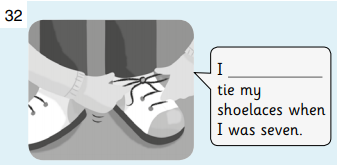*
1 point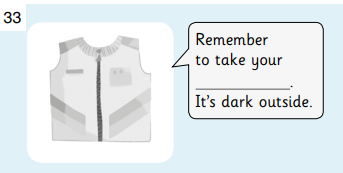*
1 point*
1 point*
1 point*
1 point*
1 point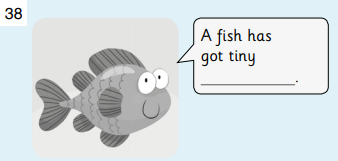*
1 point*
1 point*
1 point*
1 point*
1 point*
1 point*
1 point*
1 point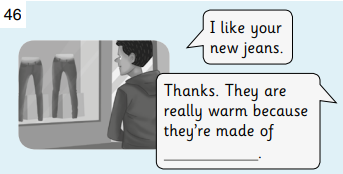*
1 point*
1 point*
1 point*
1 point*
1 point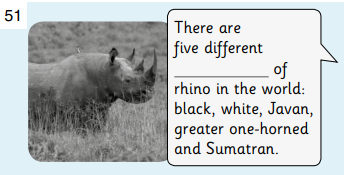*
1 point*
1 point*
1 point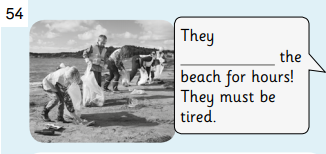*
1 point*
1 point*
1 point*
1 point*
1 point*
1 point